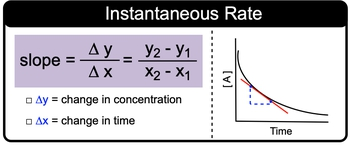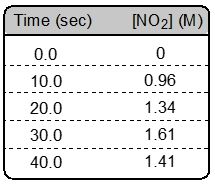## General Chemistry

Learn the toughest concepts covered in Chemistry with step-by-step video tutorials and practice problems by world-class tutors

15. Chemical Kinetics

# Instantaneous Rate

Instantaneous Rate is the rate a reaction at any particular point in time.

Instantaneous Rate
1
concept

## Instantaneous Rate Concept 11m
Play a video:
instantaneous rate is the rate of a reaction at any particular point in time. And we're going to say here that calculated using the slope of the tangent line to the curb of that point is the way we obtain our instantaneous rate. So if we're given basically a plot of Y and X coordinates, we can use that to figure out the slope which will be equal to our instantaneous rate. Now we're going to say while the rate of reaction decreases with time because the amount of reactant is decreasing in time are instantaneous rate though remains constant here, if we take a look, we say that if we're given uh points Y and X, we can figure out the slope. Remember that slope is equal to change in y over change in X rise over run this really means Y two minus Y one divided by X two minus x one. Here, we would change, we would keep the change and why as changes in our concentration and our changes in X as changes in time. Now. Here we have the graphical representation of a curve and again, we've used two points in terms of this which would relate to our tangent line. We'd utilize this formula for slope and use that to figure out our instantaneous rate. So just keep this in mind as we start investigating more and more questions dealing with calculating the instantaneous rate of an overall reaction or at any particular point2
example

## Instantaneous Rate Example 11m
Play a video: